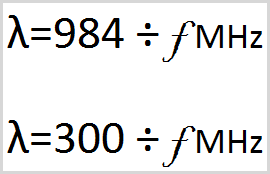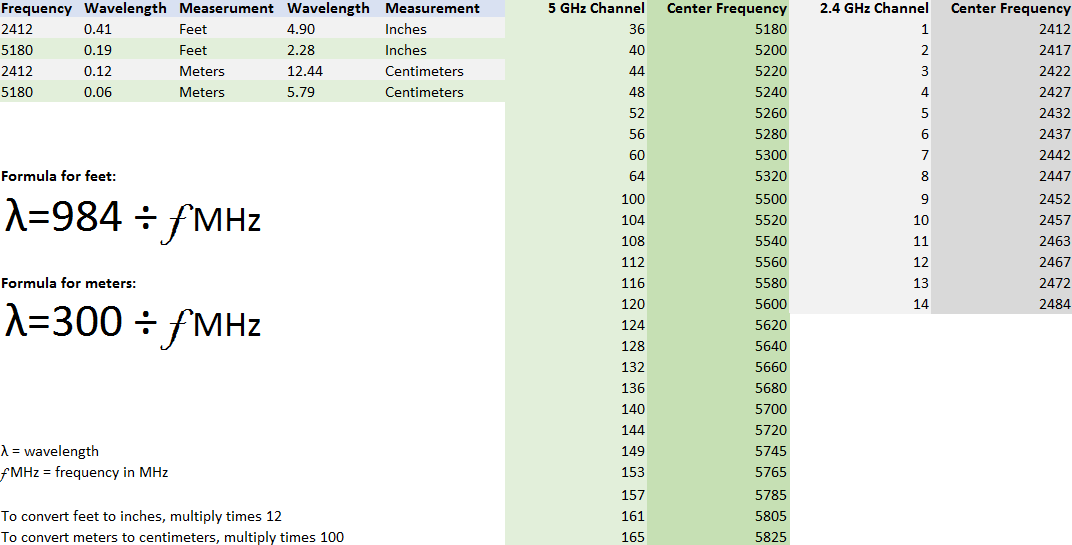# Calculating RF Wavelengths## Calculating RF Wavelengths

By Tom Carpenter On 07/25/2017 - 422 Comments

RF wavelength calculations can be made quite simple with two basic formulas. One for feet and the other for meters. This blog post provides the formulas and an Excel spreadsheet for calculating the wavelengths for each 20 MHz channel center frequency in the 2.4 GHz and 5 GHz bands.

The length of RF waves impacts antenna design decisions. Antennas should be designed so that they best receive in the frequencies intended. This means understanding the wavelength of the RF waves generated in a given frequency.

802.11 channels work on a center frequency. The image below shows the spreadsheet available here, which also shows the center frequencies for each 20 MHz channel in 2.4 GHz and 5 GHz.The common formula for calculating wavelength in feet is:

wavelength = 984 / frequency in MHz

To calculate the wavelength in meters use:

wavelength = 300 / frequency in MHz

These formulas are shown, more scientifically, in the image above. Download the spreadsheet and take a look at the result for the different center frequencies using the drop down boxes provided in the Frequency column.

Happy calculating!

Tagged with: RF, wavelength, 2.4 GHz, 5 GHz, formula

Blog Disclaimer: The opinions expressed within these blog posts are solely the author’s and do not reflect the opinions and beliefs of the Certitrek, CWNP or its affiliates.

### 0 Responses to Calculating RF Wavelengths

Subscribe by Email
<< prev - comments page 1 of 1 - next >>